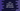# Java Arrays sort method explanation with example## Java Arrays sort method explanation with example:

In this post, we will learn java.util.Arrays.sort method with examples. This method can be used to sort the contents of an array in a given order within a range. We can provide a range optionally. If we provide a range, it will sort the items in that range.

This method also has an option to provide a comparator and we can use that to change the way the items are sorted.

In this post, we will learn how to use the sort method with different examples.

### Definition of sort:

The sort method is defined as like below:

``````sort(T[] arr, int start, int end)

sort(T[] arr, int start, int end, Comparator<? super T> comparator)``````

This method is a static method, so we can call this method directly without crating a new object of the Arrays class.

This method is defined for byte, char, double, float, int, long, Object,and short datatype.

``````sort(T[] arr, int start, int end)

sort(byte[] arr, int start, int end)

sort(char[] arr, int start, int end)

sort(double[] arr, int start, int end)

sort(float[] arr, int start, int end)

sort(int[] arr, int start, int end)

sort(long[] arr, int start, int end)

sort(Object[] arr, int start, int end)

sort(short[] arr, int start, int end)``````

Here, start and end are two optional values. If you provide these values, it will sort the array from the index start(inclusive) to end(exclusive). If both are equal, it will be an empty array.

This is a Quick sort algorithm. It uses Dual-Pivot Quicksort by Vladimir Yaroslavskiy, Jon Bentley, and Joshua Bloch. It’s time complexity is O(nlog(n)) and it is faster than the traditional (one-pivot) quicksort algorithm.

### Exceptions of sort method:

• IllegalArgumentException if start is greater than end.
• ArrayIndexOutOfBoundsException if start is less than 0 or end is greater than the length of the array.

### Example of sort:

Let’s take an example of sort:

``````import java.util.Arrays;

class Main {
public static void main(String[] args) {
int[] arr = {1, 2, 3, 9, 8, 4, 0};

System.out.println("Original array: " + Arrays.toString(arr));

Arrays.sort(arr);
System.out.println("Sorted array: " + Arrays.toString(arr));
}
}``````

Here, we are using Arrays.sort on the array arr. We are printing this array before Arrays.sort is used and after it is used.

If you run this program, it will print the below output:

``````Original array: [1, 2, 3, 9, 8, 4, 0]
Sorted array: [0, 1, 2, 3, 4, 8, 9]``````

### Example of sort with from and to:

The above example is not using any from or to index parameters. Let’s use these parameters and check how it works:

``````import java.util.Arrays;

class Main {
public static void main(String[] args) {
int[] arr = {13, 12, 11, 9, 8, 4, 0};

System.out.println("Original array: " + Arrays.toString(arr));

Arrays.sort(arr, 3, 6);
System.out.println("Sorted array: " + Arrays.toString(arr));
}
}``````

If you run this program, it will print:

``````Original array: [13, 12, 11, 9, 8, 4, 0]
Sorted array: [13, 12, 11, 4, 8, 9, 0]``````

As you can see here, it will sort the array from index 3 to index 5. 3 is included, but 5 is not.

### Example of sorting a string array:

Let’s try to sort a string array. We can use a similar approach to do that.

``````import java.util.Arrays;

class Main {
public static void main(String[] args) {
String[] arr = {"one", "apple", "boy", "cat"};

System.out.println("Original array: " + Arrays.toString(arr));

Arrays.sort(arr);
System.out.println("Sorted array: " + Arrays.toString(arr));
}
}``````

It will sort the array:

``````Original array: [one, apple, boy, cat]
Sorted array: [apple, boy, cat, one]``````

### Example of sorting a string array in reverse order:

We can pass a Comparator to sort a string array in reverse order. We can pass Collections.reverseOrder() as the second argument to Arrays.sort.

``````import java.util.Arrays;
import java.util.Collections;

class Main {
public static void main(String[] args) {
String[] arr = {"one", "apple", "boy", "cat"};

System.out.println("Original array: " + Arrays.toString(arr));

Arrays.sort(arr, Collections.reverseOrder());
System.out.println("Sorted array: " + Arrays.toString(arr));
}
}``````

It will print:

``````Original array: [one, apple, boy, cat]
Sorted array: [one, cat, boy, apple]``````

### Example of sorting an array of objects:

Let’s create an array of objects and sort these objects by using a comparator. The comparator will decide how to sort the array of these objects.

``````import java.util.Arrays;
import java.util.Comparator;

class Student {
String name;
int marks;

Student(String name, int marks) {
this.name = name;
this.marks = marks;
}

@Override
public String toString() {
return "Student{" +
"name='" + name + '\'' +
", marks=" + marks +
'}';
}
}

class StudentComparator implements Comparator<Student> {
@Override
public int compare(Student s1, Student s2) {
return s1.marks - s2.marks;
}
}

class Main {
public static void main(String[] args) {
Student firstStudent = new Student("Alex", 10);
Student secondStudent = new Student("Bob", 20);
Student thirdStudent = new Student("Chandler", 30);
Student fourthStudent = new Student("Daisy", 12);
Student fifthStudent = new Student("Emily", 60);

Student[] students = {firstStudent, secondStudent, thirdStudent, fourthStudent, fifthStudent};

System.out.println("Students array: " + Arrays.toString(students));

Arrays.sort(students, new StudentComparator());

System.out.println("Students array after sort: " + Arrays.toString(students));

}
}``````

Here,

• Student is a class to hold the info of a Student. It can store the name and marks of a student.
• StudentComparator is a class we are using as a comparator. This comparator compares the marks of two students.
• We have created five different Student objects and created one array from these objects, students.
• We are using Arrays.sort on this array and passing StudentComparator object as the second parameter.

If you run this program, it will print:

``````Students array: [Student{name='Alex', marks=10}, Student{name='Bob', marks=20}, Student{name='Chandler', marks=30}, Student{name='Daisy', marks=12}, Student{name='Emily', marks=60}]
Students array after sort: [Student{name='Alex', marks=10}, Student{name='Daisy', marks=12}, Student{name='Bob', marks=20}, Student{name='Chandler', marks=30}, Student{name='Emily', marks=60}]``````

As you can see here, after the sorting is done, it sorted the objects as per their marks. The values are printed as per the overridden toString method in the Students class.